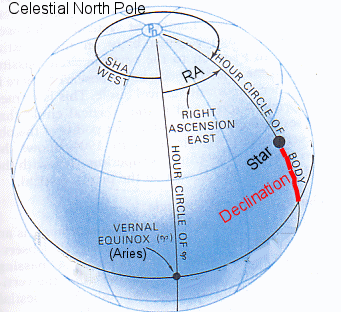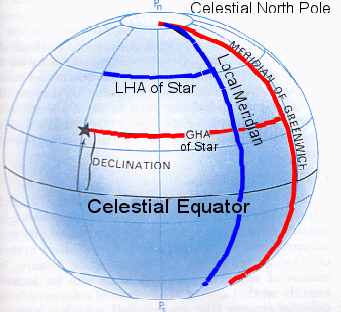# Julian Day, Julian Date, UT, GMT, GMST, LST, GHA, LHA, RA, SHA?Input Values Give Longitude Deg min sec East West Star Give Right Ascention of Star Hour Min Sec Give Greenwich Mean Time (UT) Year  Month   Day Hour  Min   Sec Mathematics Julian Day is the number of days elapsed since noon GMT on January 1st, 4713 B.C. For astronomers a day begins at noon GMT and runs until to the next noon. Here I mean UT0 at midnight. In Julian Date at UT are also hours, minutes and seconds in days. GMST is same as GHA of Aries (Vernal Equinox). GMST is calculated from UT. LST is same as LHA of Aries (Vernal Equinox).   LST = GMST + Longitude   LHA = GMST + Longitude - RA   LST = LHA + RA   SHA in degrees = 360 - RA in degrees   SHA in hours = 24 - RA in hours   GHA = GMST - RA   GHA = GMST + SHA   GHA = LHA - Longitude Astronomers uses Right Ascention (RA) RA is measured eastward from the hour circle of Aries and expressed in units of time. Nautical Almanacs use Sidereal Hour Angles (SHA). SHA is measured estward from the hour circle of Aries and expressed in units of arc. RA and SHA are equivalent.

Click on Button:

Output:
Julian Day at UT0:   Julian Date at UT:
Greenwich Mean Sidereal Time (GMST): hour min sec
Local Sidereal Time (LST): hours min sec
Local Hour Angle (LHA) of hours min sec = deg min sec
Greenwich Hour Angle (GHA) of hours min sec = deg min sec
Sidereal Hour Angle (SHA) of hours min sec = deg min sec